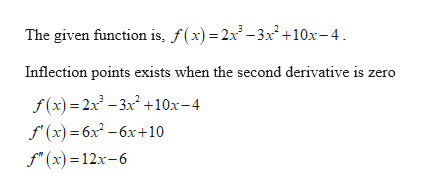# Find the inflection point(s), if any, of the functiong(x) = 2x^3 -3x^2 +10x - 4

Question
4 views

Find the inflection point(s), if any, of the function

g(x) = 2x^3 -3x^2 +10x - 4

check_circle

Step 1help_outlineImage TranscriptioncloseThe given function is, f(x) = 2x-3x +10x-4. Inflection points exists when the second derivative is zero f(x) = 2x - 3x +10x-4 f" (x) = 6x² -6x+10 f" (x) = 12x-6 fullscreen
Step 2

### Want to see the full answer?

See Solution

#### Want to see this answer and more?

Solutions are written by subject experts who are available 24/7. Questions are typically answered within 1 hour.*

See Solution
*Response times may vary by subject and question.
Tagged in

### Derivative